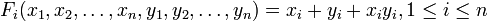# Multiplicative formal group law

## Definition

### One-dimensional multiplicative formal group law

Suppose$R$ is a commutative unital ring. The one-dimensional multiplicative formal group law over$R$ is the formal group law given by the power series:$\! F(x,y) = x + y +xy$

It is an example of a commutative formal group law.

Suppose$R$ is a commutative unital ring. The$n$-dimensional multiplicative formal group law over$R$ is the formal group law given by the following collection of power series:$\! F_i(x_1,x_2,\dots,x_n,y_1,y_2,\dots,y_n) = x_i + y_i + x_iy_i, 1 \le i \le n$

This is an example of a commutative formal group law.

## Related notions

Corresponding notion Value for one-dimensional case Value for$n$-dimensional case Comment
Lie algebra one-dimensional abelian Lie algebra over the ring$n$-dimensional abelian Lie algebra over the ring Note that for any commutative formal group law, the corresponding Lie algebra is an abelian Lie algebra. In this sense, taking the Lie algebra forgets much of the structure. In particular, the Lie algebra cannot distinguish between the additive formal group law, multiplicative formal group law, and elliptic curve group laws.
When$R$ is a field, then an algebraic group whose formal group law is this multiplicative group of$R$$n$-dimensional split torus over$R$, i.e., a product of$n$ copies of the multiplicative group.